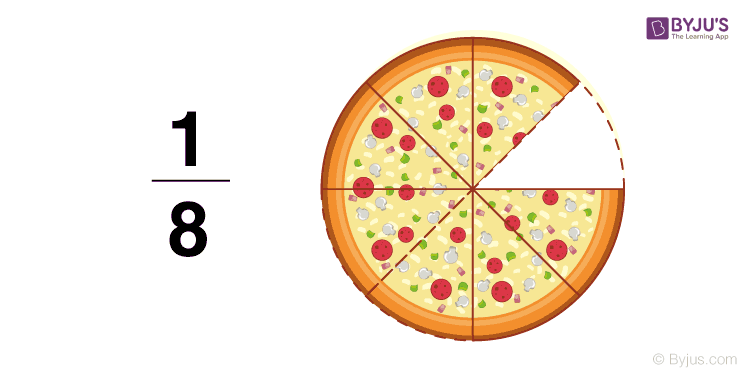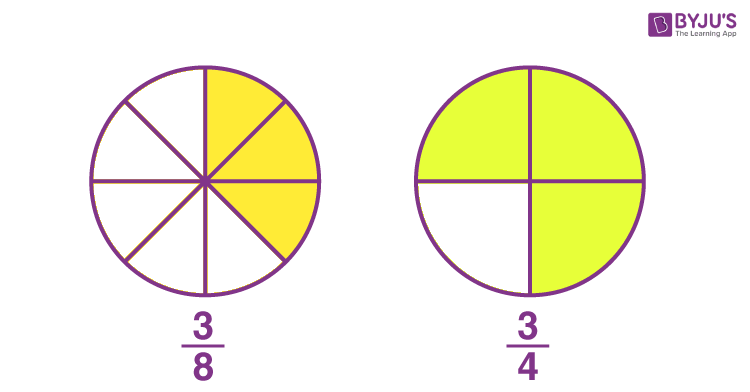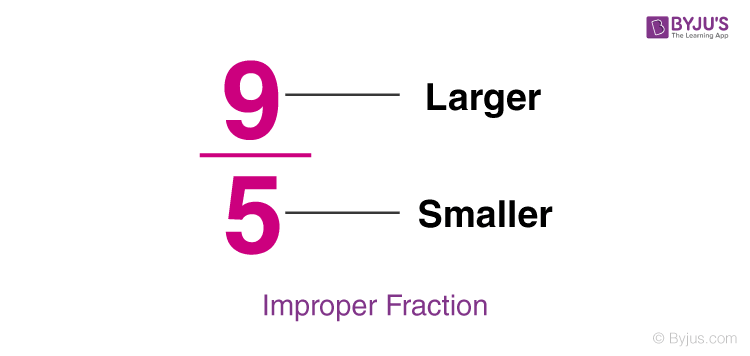Checkout JEE MAINS 2022 Question Paper Analysis : Checkout JEE MAINS 2022 Question Paper Analysis :

# Numerator

In mathematics, numerator and denominator play an essential role while dealing with fractions. As we know, that fraction represents a numerical value, which defines the parts of a whole. Suppose a number has to be divided into four parts, then it is described as p/5. So the fraction here, p/5, defines 1/5th of number p. If the line part of the fraction is at a slant, then the numerator will be on the left.

## Numerator meaning

A numerator is the part of a fraction above the line, which signifies the number to be divided by another number below the line. In the example given below, the number that lies above the line is the numerator, i.e. in the fraction 1/8, 1 represents the numerator, and 8 represents the denominator.### Numerator and Denominator

Every fraction possesses two parts, namely numerator and denominator. The upper part of the horizontal line denotes the numerator, whereas the lower part is the denominator of that fraction. The below table helps in better understanding of the numerator and denominator of a fraction.

 Fraction Numerator Denominator 7/12 7 12 (2 + 9)/ 15 (2 + 9) 15 5q/2c 5q 2c 9/(19 – 4) 9 (19 – 4) 4/3 4 3

### Numerator of a Fraction

Numerator of fraction represents the number of parts selected or removed from the total. For example, if a circle is cut into eight parts and four parts as shown in the below figure, then the selected parts of the circle, i.e. 3 over a part of the total number of parts can be written as 3/8 and 3/4, respectively.Where 3 is the numerator of the fractions 3/8 and 3/4. This number is equivalent to the shaded parts of circles given. Below are the important points about the numerator of a fraction.

• If the numerator is equal to 0, then the entire fraction becomes zero irrespective of the denominator’s value. For example, 0⁄50 is 0, 0⁄3 is 0, and so on.
• If the numerator’s value is equal to the denominator, then the fraction’s value will be equal to 1. For example, 16/16 = 1, since numerator = 16 = denominator.

### Numerator Greater Than Denominator

Not all the numerators will be less than the denominator in fractions. Sometimes the numerator will be greater than the denominator. If the numerator is greater than the denominator, then the fraction is called an improper fraction.

Example:Here, 9 is greater than 5, that means the denominator is less than the numerator.

## Numerator Examples

Example 1:

Geetha took 3 roses from a basket of 10 roses. Represent this in the form of a fraction and identify the denominator.

Solution:

Total number of roses = 10

Number of roses taken by Geetha = 3

Thus, Geetha took 3/10th of the roses from the basket.

Here, 3 is the numerator of the fraction 3/10.

Example 2:

Arun bought 20 mangoes. He gave 4 mangoes to his brother. What fraction of the mangoes was he left with? Also, find the numerator of the obtained fraction.

Solution:

Total number of mangoes = 20

Number of mangoes given to his brother = 4

Remaining mangoes = 20 – 4 = 16

The fraction of mangoes Arun was left with = 16/20 = 4/5

Thus, Arun was left with four-fifth of the mangoes.

Here, 4 represents the numerator.

## Frequently Asked Questions on Numerator

### What is a numerator and a denominator?

As we know, a fraction is the part of a whole thing. The general form of a fraction can be written as a/b, where a is called the numerator and b is called the denominator.

### What is the numerator in Division?

In division of numbers, the dividend, i.e. the number to be divided represents the numerator. For example, in the division of a number 56 by 7, i.e. 56/7, the number 56 is called the numerator

### How do you remember the numerator and denominator?

The simple way to remember the numerator and denominator is, when a fraction is given the upper part denotes the numerator, whereas the lower part denotes the denominator. For example, in the fraction 6/17, numerator = 6, denominator = 17.

### Is the numerator bigger than the denominator?

Yes, in some fractions the numerator will be bigger than the denominator, such fractions are called improper fractions. However, not in all the fractions, numerators are greater than denominators.

### Do you divide the numerator by denominator?

To divide the numerator by denominator, we need to express these parts as a multiplication of their factors. When we represent them in terms of factors, we can cancel out the common factors to get the reduced form.